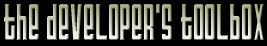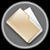This section of the archives stores flipcode's complete Developer Toolbox collection, featuring a variety of mini-articles and source code contributions from our readers.Fast Point-In-Cylinder Test   Submitted byThis function tests whether or not a 3D point lies within an arbitrarily oriented cylinder.

Collision detection systems rely on fast 3D volume primitives for their tests. Spheres and planes are the classic primitives, but these don't conform well to many models, making them inefficient & requiring the use of heavy trees or other collections. Cylinders often provide a more efficient fit to the parts of a model (especialy for character limbs!), and they can reduce the number of volume tests required.

A lightweight test for a cylinder at any orientation in space is possible. The test uses at most two dot products, a subtract, and two multiplies to determine if a point lies within the cylindrical volume. The point's distance squared from the cylinder axis is the result of the calculation, so the function is also applicable to hollow tubes. Early point-outside rejection will often happen after the first dot product.

With extra scaling based on the value of the first dot product (the 'dot' variable, related to where the point is closest to along the length of the cylinder axis) the test can be changed into a test against a cone, tapered cylinder, or elipsoid.

Enjoy, and let me know if this is useful!

-Greg J.
gjames@nvidia.com

 ```//----------------------------------------------------------------------------- // Name: CylTest_CapsFirst // Orig: Greg James - gjames@NVIDIA.com // Lisc: Free code - no warranty & no money back. Use it all you want // Desc: // This function tests if the 3D point 'testpt' lies within an arbitrarily // oriented cylinder. The cylinder is defined by an axis from 'pt1' to 'pt2', // the axis having a length squared of 'lengthsq' (pre-compute for each cylinder // to avoid repeated work!), and radius squared of 'radius_sq'. // The function tests against the end caps first, which is cheap -> only // a single dot product to test against the parallel cylinder caps. If the // point is within these, more work is done to find the distance of the point // from the cylinder axis. // Fancy Math (TM) makes the whole test possible with only two dot-products // a subtract, and two multiplies. For clarity, the 2nd mult is kept as a // divide. It might be faster to change this to a mult by also passing in // 1/lengthsq and using that instead. // Elminiate the first 3 subtracts by specifying the cylinder as a base // point on one end cap and a vector to the other end cap (pass in {dx,dy,dz} // instead of 'pt2' ). // // The dot product is constant along a plane perpendicular to a vector. // The magnitude of the cross product divided by one vector length is // constant along a cylinder surface defined by the other vector as axis. // // Return: -1.0 if point is outside the cylinder // Return: distance squared from cylinder axis if point is inside. // //----------------------------------------------------------------------------- struct Vec3 { float x; float y; float z; };float CylTest_CapsFirst( const Vec3 & pt1, const Vec3 & pt2, float lengthsq, float radius_sq, const Vec3 & testpt ) { float dx, dy, dz; // vector d from line segment point 1 to point 2 float pdx, pdy, pdz; // vector pd from point 1 to test point float dot, dsq; dx = pt2.x - pt1.x; // translate so pt1 is origin. Make vector from dy = pt2.y - pt1.y; // pt1 to pt2. Need for this is easily eliminated dz = pt2.z - pt1.z; pdx = testpt.x - pt1.x; // vector from pt1 to test point. pdy = testpt.y - pt1.y; pdz = testpt.z - pt1.z; // Dot the d and pd vectors to see if point lies behind the // cylinder cap at pt1.x, pt1.y, pt1.z dot = pdx * dx + pdy * dy + pdz * dz; // If dot is less than zero the point is behind the pt1 cap. // If greater than the cylinder axis line segment length squared // then the point is outside the other end cap at pt2. if( dot < 0.0f || dot > lengthsq ) { return( -1.0f ); } else { // Point lies within the parallel caps, so find // distance squared from point to line, using the fact that sin^2 + cos^2 = 1 // the dot = cos() * |d||pd|, and cross*cross = sin^2 * |d|^2 * |pd|^2 // Carefull: '*' means mult for scalars and dotproduct for vectors // In short, where dist is pt distance to cyl axis: // dist = sin( pd to d ) * |pd| // distsq = dsq = (1 - cos^2( pd to d)) * |pd|^2 // dsq = ( 1 - (pd * d)^2 / (|pd|^2 * |d|^2) ) * |pd|^2 // dsq = pd * pd - dot * dot / lengthsq // where lengthsq is d*d or |d|^2 that is passed into this function // distance squared to the cylinder axis: dsq = (pdx*pdx + pdy*pdy + pdz*pdz) - dot*dot/lengthsq; if( dsq > radius_sq ) { return( -1.0f ); } else { return( dsq ); // return distance squared to axis } } } ```